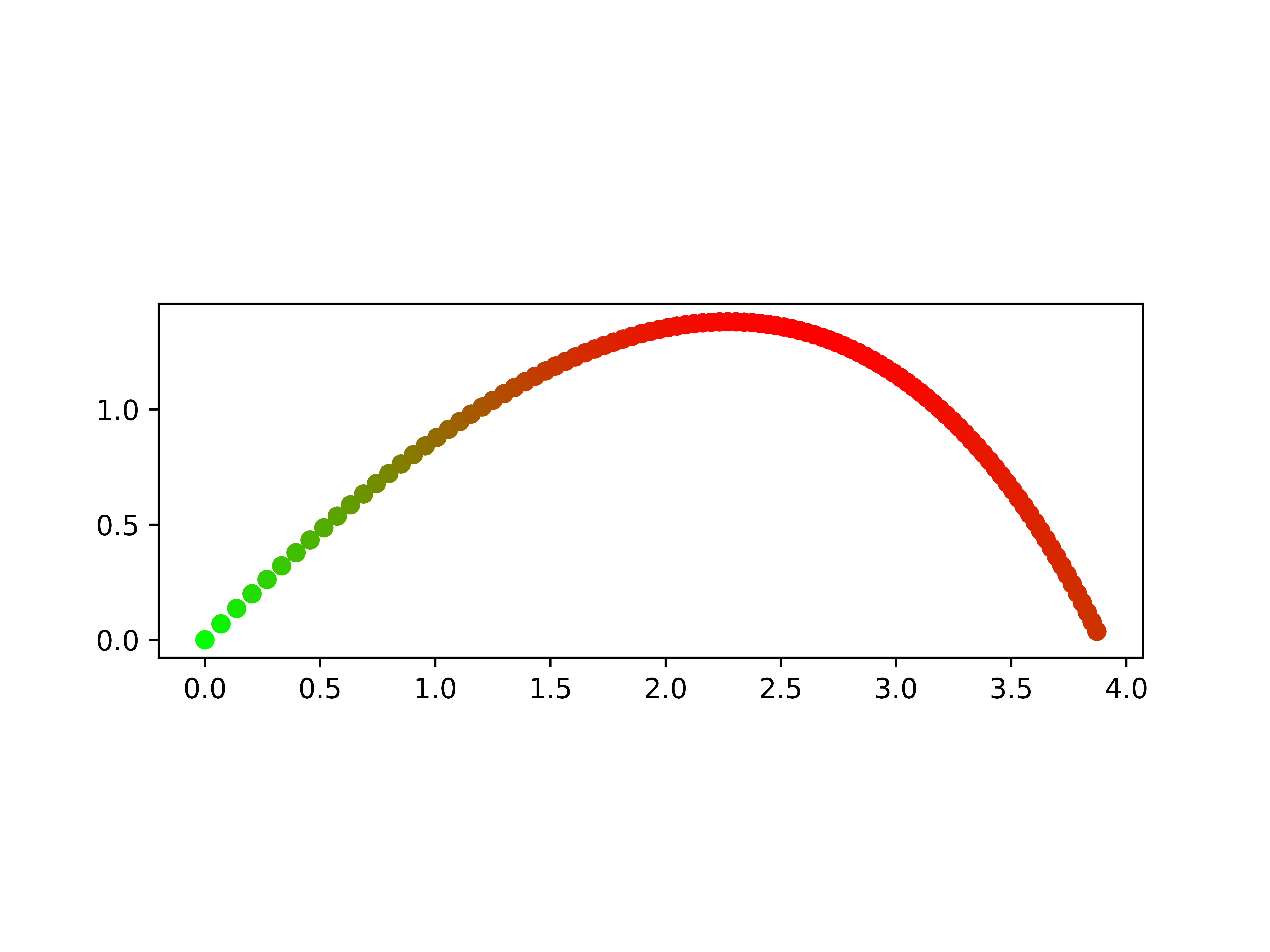A simulator for calculating the flight of a projectile in the atmosphere

Ever taken physics and wanted to not ignore air resistance well here is the program for you. It will calculate the distance a projectile will fly in the atmosphere and make a nice graph out of it:https://repl.it/@LevMckinney/Projectile-Distance-Calculator

You are viewing a single comment. View All
NathanielLowis (36)

@LevMckinney but I do have a zero division error. This is what I inputted:
launch velocity (m/s) = 100
luanch angle (deg) = 27
frontal area of projectle (m^2) = 500
drag cofficent = 2
projectle mass (kg) = 1000
Is on Earth Near Sea Level y/n : y
hight off the groud (m) = 0
time step (recomended 0.01 to 1) (sec) = .5
shold it print a plot (y/n): y
Traceback (most recent call last):
File "python", line 108, in <module>
File "python", line 98, in Distance
ZeroDivisionError: float division by zero